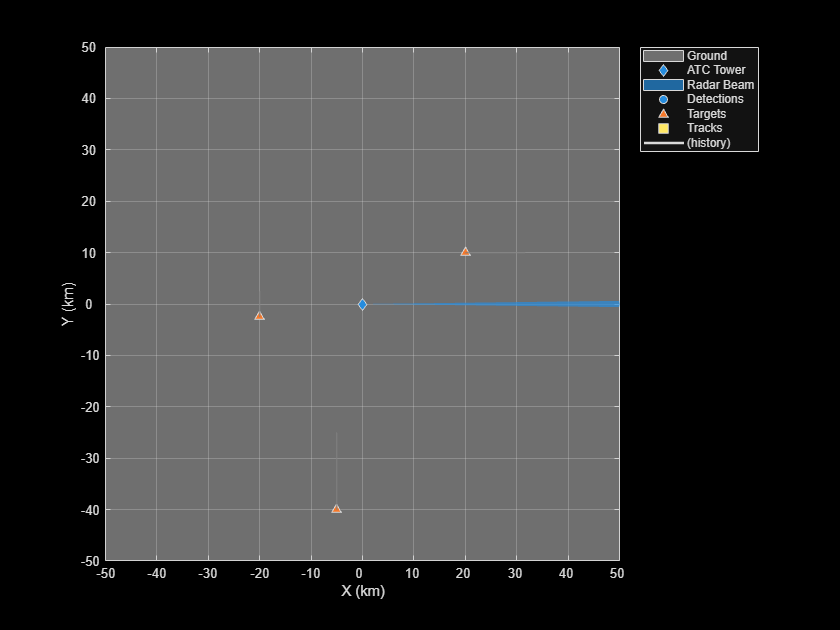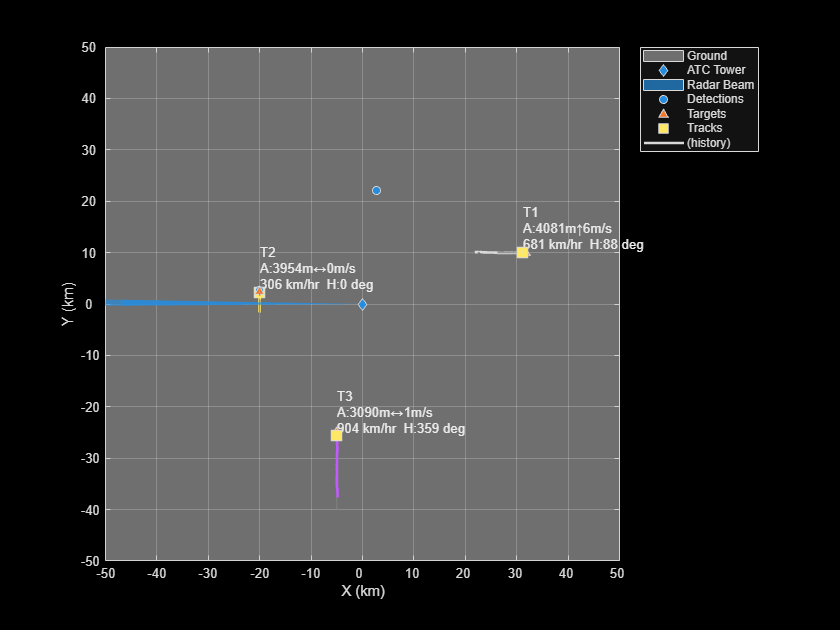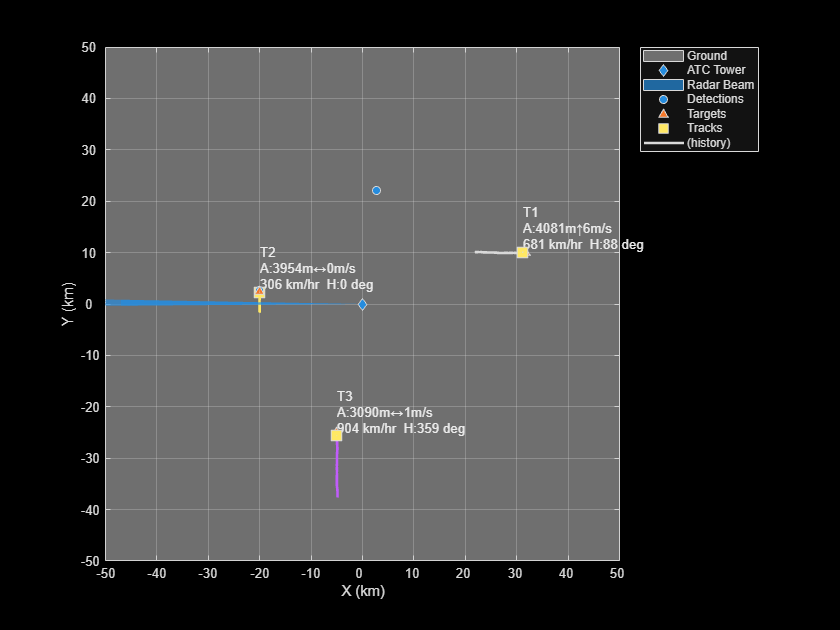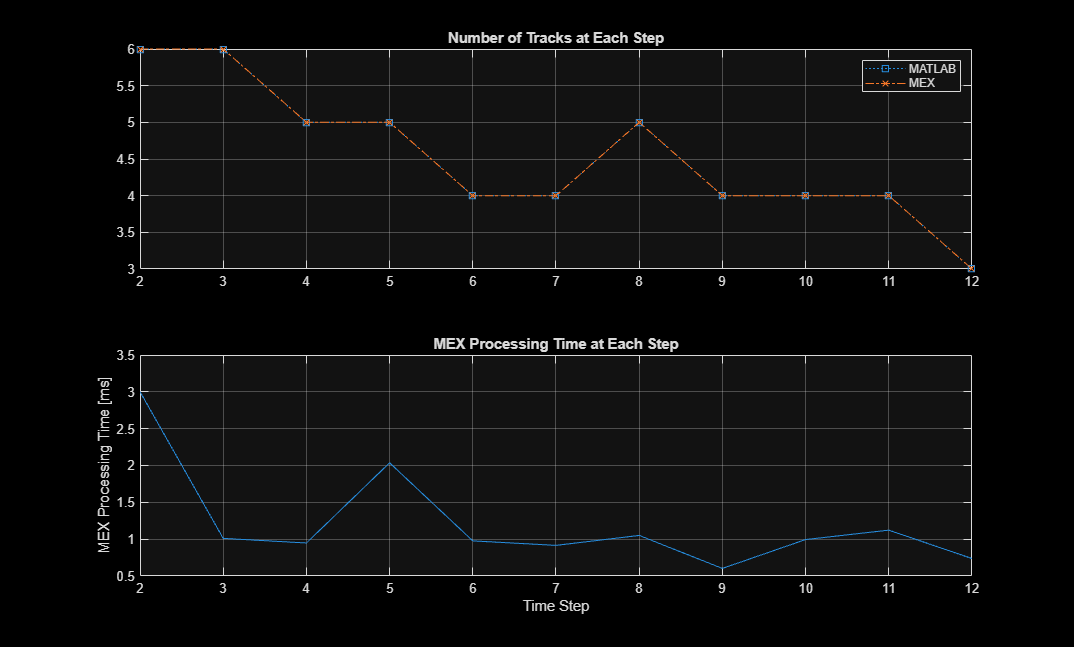# How to Generate C Code for a Tracker

This example shows how to generate C code for a MATLAB function that processes detections and outputs tracks. The function contains a `trackerGNN`, but any tracker can be used instead.

Automatic generation of code from MATLAB code has two key benefits:

1. Prototypes can be developed and debugged in the MATLAB environment. Once the MATLAB work is done, automatic C code generation makes the algorithms deployable to various targets. Additionally, the C code can be further tested by running the compiled MEX file in a MATLAB environment using the same visualization and analysis tools that were available during the prototyping phase.

2. After generating C code, you can generate executable code, which in many cases runs faster than the MATLAB code. The improved run time can be used to develop and deploy real-time sensor fusion and tracking systems. It also provides a better way to batch test the tracking systems on a large number of data sets.

The example explains how to modify the MATLAB code in the Air Traffic Control example to support code generation. This example requires a MATLAB Coder license for generating C code.

### Modify and Run MATLAB Code

To generate C code, MATLAB Coder requires MATLAB code to be in the form of a function. Furthermore, the arguments of the function cannot be MATLAB classes.

In this example, the code for the air traffic control (ATC) example has been restructured such that the `trackerGNN` that performs sensor fusion and tracking resides in a separate file, called `tracker_kernel.m`. Review this file for important information about memory allocation for code generation.

To preserve the state of the `trackerGNN` between calls to `tracker_kernel.m`, the tracker is defined as a `persistent` variable.

This function takes a cell array of `objectDetection` objects, generated by the `monostaticRadarSensor` object, and time as input arguments.

Similarly, the outputs from a function that supports code generation cannot be objects. The outputs from `tracker_kernel.m` are:

1. Confirmed tracks - A `struct` array that contains a variable number of tracks.

2. Number of tracks - An integer scalar.

3. Information about the tracker processing at the current update.

By restructuring the code this way, you can reuse the same display tools used in the ATC example. These tools still run in MATLAB and do not require code generation.

```% If a previous tracker is defined, clear it. clear tracker_kernel % Create the ATC scene with radar and platforms. [scenario,tower,radar] = helperCreateATCScenario; % Create a display to show the true, measured, and tracked positions of the % airliners. [theater,fig] = helperTrackerCGExample('Create Display',scenario); helperTrackerCGExample('Update Display',theater,scenario,tower); ```Now run the example by calling the `tracker_kernel` function in MATLAB. This initial run provides a baseline to compare the results and enables you to collect some metrics about the performance of the tracker when it runs in MATLAB or as a MEX file.

### Simulate and Track Airliners

The following loop advances the platform positions until the end of the scenario. For each step forward in the scenario, the radar generates detections from targets in its field of view. The tracker is updated with these detections after the radar has completed a 360 degree scan in azimuth.

```% Set simulation to advance at the update rate of the radar. scenario.UpdateRate = radar.UpdateRate; % Create a buffer to collect the detections from a full scan of the radar. scanBuffer = {}; % Initialize the track array. tracks = []; % Set random seed for repeatable results. rng(2020) % Allocate memory for number of tracks and time measurement in MATLAB. numSteps = 12; numTracks = zeros(1, numSteps); runTimes = zeros(1, numSteps); index = 0; while advance(scenario) && ishghandle(fig) % Generate detections on targets in the radar's current field of view. [dets,config] = detect(scenario); scanBuffer = [scanBuffer;dets]; %#ok<AGROW> Allow the buffer to grow. % Update tracks when a 360 degree scan is complete. if config.IsScanDone % Update tracker index = index + 1; tic [tracks, numTracks(index), info] = tracker_kernel(scanBuffer,scenario.SimulationTime); runTimes(index) = toc; % Gather MATLAB run time data % Clear scan buffer for next scan. scanBuffer = {}; end % Update display with current beam position, buffered detections, and % track positions. helperTrackerCGExample('Update Display',theater,scenario,tower,scanBuffer,tracks); end ```### Compile the MATLAB Function into a MEX File

Use the `codegen` function to compile the `tracker_kernel` function into a MEX file. You can specify the `-report` option to generate a compilation report that shows the original MATLAB code and the associated files that were created during C code generation. Consider creating a temporary directory where MATLAB Coder can store generated files. Note that unless you use the `-o` option to specify the name of the executable, the generated MEX file has the same name as the original MATLAB file with `_mex` appended.

MATLAB Coder requires that you specify the properties of all the input arguments. The inputs are used by the tracker to create the correct data types and sizes for objects used in the tracking. The data types and sizes must not change between data frames. One easy way to do this is to define the input properties by example at the command line using the `-args` option. For more information, see Input Specification (MATLAB Coder).

```% Define the properties of the input. First define the detections buffer as % a variable-sized cell array that contains objectDetection objects. Then % define the second argument as simTime, which is a scalar double. dets = coder.typeof(scanBuffer(1), [inf 1], [1 0]); compInputs = {dets scenario.SimulationTime}; % Code generation may take some time. h = msgbox({'Generating code. This may take a few minutes...';'This message box will close when done.'},'Codegen Message'); % Generate code. try codegen tracker_kernel -args compInputs; close(h) catch ME close(h) delete(videoDisplayHandle.Parent.Parent) throw(ME) end ```

### Run the Generated Code

Now that the code has been generated, run the exact same scenario with the generated MEX file `tracker_kernel_mex`. Everything else remains the same.

```% If a previous tracker is defined, clear it. clear tracker_kernel_mex % Allocate memory for number of tracks and time measurement numTracksMex = zeros(1, numSteps); runTimesMex = zeros(1, numSteps); % Reset the scenario, data counter, plotters, scanBuffer, tracks, and rng. index = 0; restart(scenario) scanBuffer = {}; clearPlotterData(theater); tracks = []; rng(2020) while advance(scenario) && ishghandle(fig) % Generate detections on targets in the radar's current field of view. [dets,config] = detect(scenario); scanBuffer = [scanBuffer;dets]; %#ok<AGROW> Allow the buffer to grow. % Update tracks when a 360 degree scan is complete. if config.IsScanDone % Update tracker. index = index + 1; tic [tracks, numTracksMex(index), info] = tracker_kernel_mex(scanBuffer,scenario.SimulationTime); runTimesMex(index) = toc; % Gather MEX run time data % Clear scan buffer for next scan. scanBuffer = {}; end % Update display with current beam position, buffered detections, and % track positions. helperTrackerCGExample('Update Display',theater,scenario,tower,scanBuffer,tracks); end ```### Compare the Results of the Two Runs

Compare the results and the performance of the generated code vs. the MATLAB code. The following plots compare the number of tracks maintained by the trackers at each time step. They also show the amount of time it took to process each call to the function.

```figure(2) subplot(2,1,1) plot(2:numSteps, numTracks(2:numSteps), 's:', 2:numSteps, numTracksMex(2:numSteps), 'x-.') title('Number of Tracks at Each Step'); legend('MATLAB', 'MEX') grid subplot(2,1,2) plot(2:numSteps, runTimesMex(2:numSteps)*1e3); title('MEX Processing Time at Each Step') grid xlabel('Time Step') ylabel('MEX Processing Time [ms]') ```The top plot shows that the number of tracks that were maintained by each tracker are the same. It measures the size of the tracking problem in terms of number of tracks. Even though there were 3 confirmed tracks throughout the tracking example, the total number of all tracks maintained by the tracker varies based on the number of tentative tracks, that were created by false detections.

The bottom plot shows the time required for the generated code function to process each step. The first step was excluded from the plot, because it takes a disproportionately longer time to instantiate all the tracks on the first step.

The results show the number of milliseconds required by the MEX code to perform each update step on your computer. In this example, the time required for the MEX code to run an update step is measured in a few milliseconds.

### Summary

This example showed how to generate C code from MATLAB code for sensor fusion and tracking.

The main benefits of automatic code generation are the ability to prototype in the MATLAB environment, and generate a MEX file that can run in the MATLAB environment. The generated C code can be deployed to a target. In most cases, the generated code is faster than the MATLAB code, and can be used for batch testing of algorithms and generating real-time tracking systems.